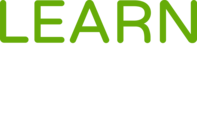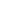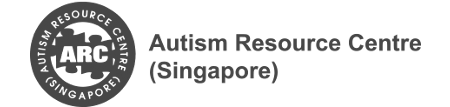#Mathematics: Lever Up is a Course

## Mathematics: Lever Up

Started 16 Mar 2020

### Full course description

This series is for students to revisit a few essential ideas in the topics of number operations, including whole numbers, fractions, and decimals.

It also helps educators understand the essential ideas for these topics so that students who struggle with mathematics will receive the necessary emphasis to meet the requirements of the national curriculum.

This course will cover the following topics:

2. multiplication facts
3. multi-digit calculations
4. fractions and decimals

This course contains four modules:

• Multiplication Facts: Use Addition to Multiply
• Multiplication Facts: Use Double to Multiply
• Multiplication Facts: Use Subtraction to Multiply

To recall the essential ideas in the topics of number operations, including whole numbers, fractions, and decimals.

This is an online course for Pathlight School students. To take this course, you’ll need to be a student of Pathlight School.

Pathlight School students can enrol in this course after logging in to Learn for Life eCampus.## Dr Yeap Ban Har

Director of Curriculum, Pathlight School

### Offered Byacademics, academic-bridging, math, mathematics, literacy-and-numeracy, numeracy, math-concepts, mathematics-concepts, primary-math, primary-mathematics, primary, lower-primary, upper-primary basic,